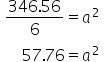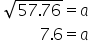Maths-
General
Easy

Question

# Find out the side of cube if the complete surface area is given to be 346.56 cm2.

## 6.77.65.68.6Hint:

## The correct answer is: 7.6

### Step 1:We have given the total surface area of the cube. Area = 346.56 cm2 Step 2: We know that Surface Area = 6a2                                                                               346.56 = 6a2 ·          Step 3: For finding the side firstly divide both sides if equation by 6Step 4:- Taking square root of both sides we get,·          Step 5: Therefore, the length of side of the given cube is 7.6 cm2. Therefore, the correct answer is option B) 7.6 cm.#### With Turito Foundation.#### Get an Expert Advice From Turito.